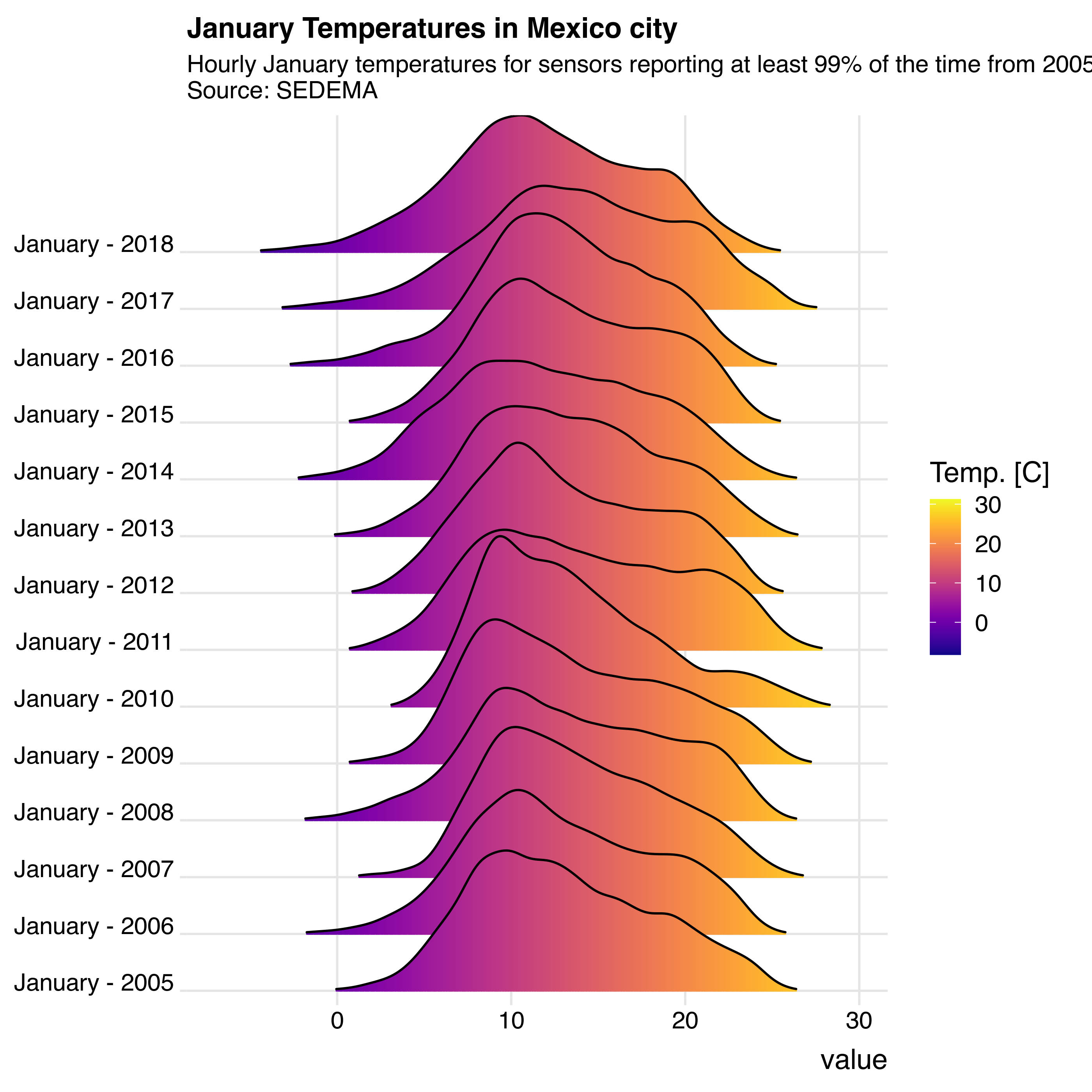Install the necessary packages:

# based on https://cran.r-project.org/web/packages/ggjoy/vignettes/gallery.html
## Auto-install required R packages
if (!require("pacman")) install.packages("pacman")
pacman::p_load(aire.zmvm, dplyr, ggplot2, tidyr, viridis, stringr, lubridate,
ggridges, purrrlyr)

# Download January TMP from 2005 to 2008
# You could get TMP data accurate to one digit by using the get_station_data
# but there's no data for 2018 that way
temp <- data.frame()
for (year in 2005:2018) {
df2 <- get_station_month_data("HORARIOS", "TMP", year, 1)
temp <- rbind(temp, df2)
}

Clean the data and only use stations that were reporting 99% percent of the time during the period under analysis.

# remove stations that always report 0
temp <- temp %>%
mutate(year = year(temp$date)) %>% group_by(year, station_code) %>% filter(!sum(value, na.rm = TRUE) == 0) %>% ungroup() # Which stations reported a temperature value at least 99% of the time reporting_stations_99 <- temp %>% group_by(year = year(date), station_code) %>% summarise(per = sum(!is.na(value)) / length(station_code)) %>% filter(per > .99) %>% select(year, station_code) %>% slice_rows("year") %>% by_slice(function(x) unname(unlist(x)), .to = "vec") # Subset only those stations that reported 99% of the time from # 2005 to 2018 temp <- filter(temp, station_code %in% unique(do.call(c, reporting_stations_99[]))) print(unique(do.call(c, reporting_stations_99[]))) ##  "CES" "MON" "PED" "PLA" "TLA" "VIF" "XAL" "FAC" "MER" "TAC" "TAH" ##  "TPN" "CUA" "SAG" "IMP" "ACO" "SUR" "SFE" "CHO" "CUT" "AJM" "MGH" ##  "NEZ" "UAX" "UIZ" "AJU" "MPA" "BJU" "SS1" Finally we plot the data: temp$month <- months(temp$date) temp$month <- factor(temp$month, levels = rev(unique(temp$month)) )
temp$month <- str_c(temp$month, " - ", year(temp\$date))

ggplot(temp, aes(x = value, y = month, fill = ..x..)) +
theme_ridges(font_size = 13, grid = TRUE) + theme(axis.title.y = element_blank())
## Picking joint bandwidth of 0.746